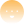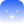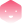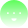LearnUseBuild
use

# Tinlake Financial Concepts

Contributors## Interest Rate Methodology

Tinlake uses an interest rate mechanism that is typically implemented as compounding per second. The implementation can be found in github.com/centrifuge/tinlake-math.

Below we show abstract examples of how this is calculated:

Variable
Description
$P$
Principal
$D$
Debt
$rate$
interest rate per second
$n$
the number of times the interest is compounded, compounding is once per second, so "n" is seconds past since last calculation
$mathtt{R}$
Nominal interest rate (5% would be 0.05)
$mathtt{A}$
Annual Percentage Rate (APR)
$mathtt{y}$
constant, seconds in a year: $3600 cdot 24 cdot 365 = 31536000$

The basic formula is:

$D = P cdot rate^{n}$

Debt equals to Principal (or the previous debt amount) times rate to the power of seconds since last calculation.

### Example: Interest rate compounding per second

$P = 100 ewline r = 0.05 ewline n = 3600 cdot 24 cdot 365 = 31536000 ewline$

Using the formula above, the Debt $D$ after half a year $(n = mathtt{y} / 2 = 15768000)$ would result in $D = 102.5315$.

After one year ($n = mathtt{y}$) the $D$ would be $105.1271$.

Thus a 5.00% interest rate compounded every second is equivalent to an annually compounded rate of 5.127%.

The annual percentage rate $mathtt{A}$ could also be calculated directly from the percentage rate $mathtt{R}$ (using $n = mathtt{y} = 31536000$):

$mathtt{A} = (1 + rac{mathtt{R}}{mathtt{y}})^mathtt{y} = 1.05127$

#### Rate per Second

To calculate the Debt, we initialize an interest rate in Tinlake with a variable called ratePerSecond or $rate$. The ratePerSecond represents the interest accrued per second in Tinlake.

$rate = 1 + rac{mathtt{R}}{mathtt{y}}$

#### Calculate Debt

$D = P * rate^t$

The debt can be calculated by multipling the principial $P$ with $rate$ to the power of $n$. The variable $n$ represents the time passed in seconds since the loan has been borrowed.

Continuing the example from above for annual interest:

$rate = 1 + rac{0.05}{31536000} = 1.0000000015854900 ewline D = 100 cdot 1.0000000015854900^{31536000} = 105.1271$

### Using an annual percentage rate (APR) in Tinlake

The Tinlake User Interface uses an annual percentage rate (APR) as input. Tinlake transforms this annually compounded rate $mathtt{A}$ into the equivalent rate used for compounding per seconds $rate$. This is achieved by solving the equation:

$mathtt{A} = (1 + rac{rate}{mathtt{y}})^mathtt{y}$

for $r$:

$r = mathtt{y} cdot (mathtt{A}^{ rac{1}{mathtt{y}}}-1)$

Using the calculated $r$ compounding every second leads to the same amount of debt like using $mathtt{A}$ compounding annually over the course of a year. Thus, the calculated rate can be used to achive an interest per year (APR) behaviour with the compounding per second implementation in Tinlake.

Continuing the example from above with an 5.00% annual interest rate (APR):

### Decimal Precision

We use fixed precision decimals for any monetary amounts. Interest Rates are typically of type ray with 27 digits precision and amounts are of type wad which has 18 digits precision.

This is usually explicitally mentioned in throught the codebase.

## Asset Pricing

Centrifuge’s Tinlake is an asset-backed smart contract lending platform built to responsibly bridge real-world assets, such as invoices, mortgages or streaming royalties to the DeFi ecosystem. This post is the first in a multiple part series about how valuation and pricing of real-world assets work within the Tinlake ecosystem. Collectively, this series will form our knowledge base on real-world asset pricing and will be used to educate the DeFi community as we bridge non-native crypto assets into the decentralized finance ecosystem. This post starts with the fundamentals of pricing real-world assets. It will focus on one particular asset — an invoice — and explain which factors are usually considered in pricing this asset and how these factors may be turned into an interest rate. Invoices are particularly interesting collateral for Tinlake and DeFi because they have historically low default rates and a short maturity of 30–90 days.

### Financing an Invoice

For our purposes, an invoice is a bill sent from one entity (supplier) to another (buyer) in connection with the delivery of goods or services. Key data points on an invoice usually are:

1. Invoice amount
3. Issuance date
4. Due date
5. Line items (e.g. type and quantity of goods)
6. Payment terms and conditions

Suppliers around the world typically have to wait for 30–90 days to get paid for an invoice. Instead of waiting several months until the invoice is due, the supplier has an alternative: find a way to convert the invoice into capital quickly by financing it through a capital provider, e.g. an Asset Originator or a factor.

The parties involved in issuing and financing an invoice through Tinlake usually are the following:

Roles
Description
Supplier/Borrower
Invoices the buyer for delievered goods or services; has to wait for payment by the buyer; finances this invoice to get paid right away.
Purchases the goods or services and incurs the charges related to the invoice for the goods or services
Asset Originator
Provides capital to supplier at a discount to the face value of the invoice, and 100% of the collections related to the invoices.
Lender
Lends money to the Asset Originator in exchange for certain rights and privileges regarding the invoice payments of buyers.

Financing an invoice can be structured as a simple loan with one bullet repayment. The two decisive pricing parameters are advance rate and interest rate. The advance rate acts as a reserve or protection for the Lender against losses. The interest amount/finance fee is usually deducted up-front and the loan is then paid back in relation to the payment due date of the invoice.

Imagine e.g. an invoice with a face value of USD 100 due in 90 days. A supplier may use this invoice as collateral to receive early payment in form of a short term loan. Assuming an 80% advance rate and a 7.0% interest rate (APR) a supplier could receive a loan (“early payment amount”) of USD 78.6 (deducting interest of USD 1.4 = USD 80 (7.5% (90/360)) and pay back USD 80 after 90 days when he receives the payment of the buyer. If the supplier wants to finance this invoice through Tinlake, he could turn to an Asset Originator who would tokenize it and use a Tinlake pool to draw funding in stablecoin from a Lender, e.g. from a decentralized lending protocol, against the invoice NFT as collateral.

### Factors for Pricing Invoices

There are several different factors to consider when setting advance rate and fee/interest rate for financing an invoice. These different factors are relevant for both the Asset Originator as well as the Lender. For brevity, I will refer to both parties as just the Lender in the section below:

The value of an invoice is firstly based on the buyer’s ability and willingness to pay. In some cases, this can be represented by publicly available credit ratings. In others, credit bureau data, the payment history of the buyer or publicly available news may be used.

#### 2. Supplier Credit Risk

The stability of the supplier is another important factor to be considered. Lenders are underwriting the supplier as much as the invoices themselves. The suppliers working capital management is of particular importance. Is the supplier’s net working capital positive or negative? How have its metrics trended in the past? What is the supplier’s collection history with this specific buyer? Also, what is its history of commercial disputes and/or failures to deliver?

#### 3. Industry and Country

In addition to the supplier’s and buyer’s individual credit risk, the market impact on pricing by industry and country is another important factor. While some industries have a robust, constant supply chain, other industries may be subject to seasonality or prone to disruption. Each country carries with it some amount of systematic risk that trickles down to suppliers and its ability to pay. Further, the recovery of defaulted amounts or the ability to collect an overdue payment often depends on a country’s legal system.

#### 4. History

There is inherent bias baked into repeating relationships that can be either positive or negative. If a Lender has some track record with a supplier/borrower, then the impact of a continuing relationship will work its way into the pricing. For instance, if an efficiently run supplier has a long track record, with invoices based on contracts with stable counterparties, they will get favorable pricing on its invoice population.

#### 5. Portfolio Volume

The most important factor when estimating the advance rate/fee for invoices is volume. To a Lender, a large number of invoices means the risk of non-payment is spread across a number of counterparties — more invoices are better than less. If one is pricing a pool of invoices, the statistics around the invoice pool can be considered. One can think of a distribution function where a great sample amount means a smoother distribution. Lower invoice counts mean that collecting on the discrete, single invoice has a more significant impact on the pool’s return.

### Estimating Advance Fee and Rate

Now let’s take a look at how considering all these factors may translate into advance rate and a fee/interest rate for financing an invoice. This will be illustrated with a very simple example. Having this in mind, please note that valuation and pricing generally come with a large variety of approaches and different, usually higher levels of complexity.

Imagine a large Buyer, “Sneaker Group” and a Supplier, “Laces Inc”. There is also “Lender Co” willing to provide financing. Laces invoices Sneaker for its delivery of laces worth USD $1,000 with payment due in 90 days. Laces wants to request financing from Lender with the invoice as collateral. To set interest and advance rates, the Lender may use a homemade risk scorecard. These scorecards come in a variety of forms and different levels of complexity. For this purpose, we will assume a very simple scorecard, where with five individual factors described above (Note, that portfolio aspects are not considered for this simple example of an individual invoice) contribute equally with a score from 1 (the worst) to 10 (the best) to an overall risk score of up to 50. Let’s assume the following scores for Laces Inc: Factor Score Description Supplier Credit Worthiness ("Laces, Inc.") 7 Laces provides its financial information, tax returns, bank statements to AO so that it can estimate credit worthiness. The analysis leads to an above average score which implies risk of default is lower compared to the overall market. Lower advance rates needed. Buyer Credit Worthiness (“Sneaker Group”) 10 Sneaker is a large buyer and publicly traded with excellent credit ratings provided by established rating agencies Relationship/History 7 A strong relationship means less variance around the outcome, thus lower risk. Industry 5 Lace’s industry is cyclical and can impact collections. Country 7 Sneaker and Laces operate lower risk jurisdictions and warrant pricing that reflects this lower risk. Adding up the individual scores for each of these factors leads to a risk score of 36 for Laces. This risk score can be mapped on a risk scorecard with assigned advance and interest rates for risk scores. The typical advance rate is usually between 70% to 90%. As of the writing of this blog, annual rates (APR) around 6% to 8% on a loan 90-day invoice are consistent with observable market rates. These rates would also include and reflect the Lenders operational costs and cost of capital, which are similar for all loans. Having this in mind, a simple scorecard could look like this: Risk Rating Score Advance Rate Interest Rate A 45-50 90% 5.00% B 40-44 80% 6.00% C 30-39 80% 7.00% D 20-29 70% 8.00% F 5-20 No financing approved ("Risk cut-off") Based on this scorecard, Laces would be assigned a risk rating of C and Lender could offer Laces financing with an advance rate of 80% and an interest rate/fee of 7.00% against the invoice as collateral. The Asset Originator would advance$786 (80% advance less $14 interest at 7% interest) and collect$800 in 90 days from Laces.

## Asset Valuation

### Intro

Asset Valuation is the process of determining the current worth of an asset or portfolio by assigning a monetary value. The value of a portfolio of assets is often also expressed as the net asset value (NAV).

A NAV is usually required when a portfolio is sold or when investors want to join/exit an existing investment pool. Then the portfolio value ultimately determines the investment/redemption price. Note that for these purposes the portfolio value may be different to the book value or accounting value of a portfolio.

Determining the value of illiquid assets is notoriously difficult because – by definition – there isn’t a liquid secondary market to determine the value, unlike many stocks, bonds or most fungible tokens. For illiquid asset portfolios the valuation methodology is thus often based on a fair value valuation utilizing a financial model ("marked to model"). This often comes down to valuing the present value of future cash flows expected to receive based on these financings - the so-called discounted cash flow (“DCF”) method.

### Tinlake's approach - Fair value DCF

#### Step-by-step overview

Tinlake's valuation methodology is also based on a fair value valuation ("marked to model") utilizing a discounted cash flow model. The approach can be summarized as follows:

1. Derive Expected Cash flows For every outstanding financing of an asset, the expected cash flow is calculated. The current implementation allows to calculate the Expected Repayment of simple bullet loan structures which are common in particular in invoice financing and trade finance. The Expected Cash Flow is calculated based on (i) the expected repayment dates and (ii) the expected repayment amounts. (i) The expected repayment date is derived on contractual obligations associated with the financing, e.g. the due date of the underlying invoice. This is provided through an Oracle based on the documents underlying the NFT minted on Centrifuge's P2P Protocol. (ii) The expected repayment amount is projected based on the outstanding Tinlake financing by applying the financing fee on the current debt until the repayment date.

2. Risk-adjust expected cas flows The expected Cash Flow is risk-adjusted for credit risk by the Expected loss. Every financing is allocated a risk class that has a Probability of Default (PD) and Loss Given Default (LGD) assigned to it. The Expected Loss is calculated as Expected loss = Expected Cash Flow * PD * LGD and substracted from the expected repayment amount to adjust for credit risk. Note that PDs are often communicated per anno and may need to be adjusted to the term of the underlying asset.

3. Discount risk-adjusted expected cash flows The risk-adjusted expected cash-flows are discounted with an appropriate discount rate (this depends on asset class and pool) to derive the present value of a financing. The discount rate usually reflects the rate of return an investor could earn in the marketplace on an investment of comparable size, tenor and risk. Note, that the discount rate is the same for every financing of a pool. The standard formula to calculate the PV of a cash flow is

$PV= rac{CF}{(1+r)^t}$

with r = discount rate and t = period of cash flows. As we deal with intra-year cash flows, the formula becomes

$PV= rac{CF}{(1+ rac{r}{n})^{t*n}}$

with $n$ being number of discounting periods per year (e.g. 360 days for a financial year).

1. Calculate NAV Adding up the present values of the risk-adjusted expected cash flows for all financings in the pool leads to the (portfolio) NAV. The NAV plus the liquidity currently in the Reserve of the Pool gives the Pool Value.#### Write-offs

Tinlake allows for a flexible treatment of write-offs. If a financing is overdue the expected repayment amount can be (partially) reduced by defined percentages after a defined number of days following pre-determined criteria (e.g. a grace period and collection period).

#### Operational costs

Average loan maintainance/running costs (such as legal, SPV, servicing) could be substracted from the PV. At the moment these are set to zero in Tinlake's NAV calculation as operating costs are currently borne by the SPV of the issuer.

### Sample calculations

#### Simple example for one financing

General Assumptions: Today = 31.03.2020 Discount rate r = 5.00% Days per year: 360 Seconds per year: 31536000

Financing parameters: Financing date = 01.01.2020 Financing amount = 100 DAI Financing fee = 10% APR Expected repayment date = 30.06.2020 Expected loan duration = 180 days (Annual) PD = 4% LGD = 50%

Calculations

1. Calculate the expected cash flow on the 30.06.2020

Remember the textbook compounding formula is

$Expected CF = P * (1 + rac{r}{n})^{(n*t)}$

where P = Principal in DAI [=100] r = Interest rate (decimal [0.1]) t = Time --> Loan duration i years n = Number of times interest is compounded per unit t

Applying this to our financing assuming compounding per second gives P = 100 DAI r = 0.1 t = loan duration in years = 180/360 days = 0.5 n = 31536000 seconds per year

$Expected CF = 100 DAI * (1 + rac{0.1}{31536000})^{(31536000* rac{180}{365})} = 105.13 DAI$
1. Adjust the expected cash flow for default risk:

The Expected Loss with the risk parameters given is

$Expected Loss = 105.13 DAI * (0.04/180*360) * 0.5 = 1.05 DAI$

Note that the PD is adjusted to reflect the term of the asset. This is substracted from the Expected CF to calculate the risk-adjusted expected CF: 105.13 DAI - 1.05 DAI ~ 104 DAI

1. Discount the risk adjusted expected Cash Flow Assuming that today is the 31.03.2020 we first calulate the remaining time till the expected cash flow (30.06.2020) which is 90 days. Applying the discounting formula above with r = 0.05 t = 90 / 360 = 0.25 = loan duration in years n = 31536000 seconds per year gives:
$PV = rac{104 DAI}{(1 + rac{0.05}{31536000})^{0.25*31536000}} = 101.5 DAI$